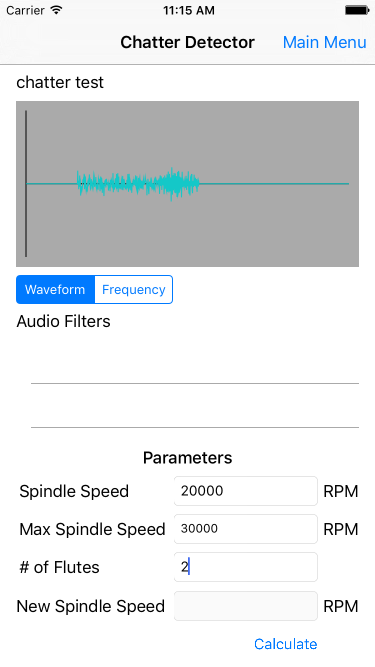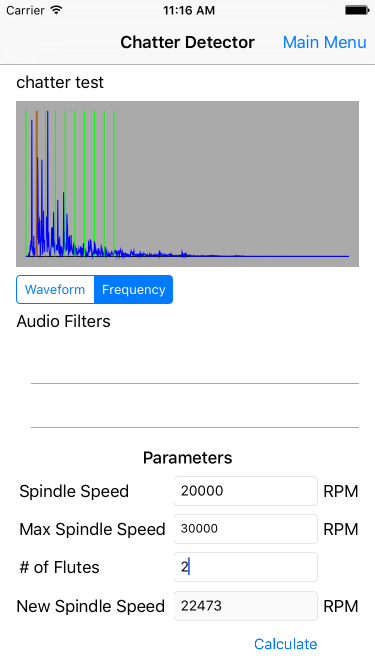# Milling Chatter Detection App

My partner in crime for the SME Sucros3D project, Stephanie, created a Matlab Chatter Detection app for her Senior Design project. The original goal was to create a functioning iOS version because Professor Soshi is an apple user. Unfortunately, many Mechanical Engineering students do not have an extensive programming background so they were unable to navigate the iOS SDK and implement the project. I reached out to Professor Soshi to see if I could try my hand at developing the app. It would consist of taking their MatLab program and essentially converting it to Swift. The app reads in a recorded audio file. It then generates FFT plots and finds the chatter frequency based on some nifty math.

#### GUIJan 27, 2017

This is the GUI I designed for the app. I still need to implement the filters sections of the app and hopefully a profile save feature (CoreData).#### Generating FFT DataApr 01, 2016

The hardest part of this endeavor is understanding how to process audio data in iOS. Opening the audio file to extract the waveform was very frustrating because there were a bunch of different parameters that I did not understand. At the end I ended up with using a PCM buffer of sorts.

The next part is generating the FFT data, this is where Stephanie got hung up. I decided to attack this using Apple's Accelerate framework. This framework is written in Obj-C++ so my C++ background with pointers and what not came in handy. It took me awhile to understand how to pass different variables and call the appropriate functions to generate the FFT. I compared the results of my FFT with the MatLab version and I was not getting the same values. After spending several days I realized that Matlab does some kind of scaling. In the end my FFT data was valid. Figuring out this part is probably going to be the hardest part of the project and it's times like these that remind you how easy we have it when we use modern languages like Swift, Python, and MatLab. Hopefully one day I'll add some markup to format code better.

DSPSplitComplex fft(NSArray *dataInput, const int log2n){ const int n = 1 << log2n; const int nOver2 = n/2; float *input; input = malloc(n * sizeof(float));

``````FFTSetup fftSetup = vDSP_create_fftsetup (log2n+1, kFFTRadix2);

int i;
for(i = 0; i < n; i++){
input[i] = [(NSNumber *)[dataInput objectAtIndex:i] floatValue];
}

DSPSplitComplex fft_data;
fft_data.realp = malloc(nOver2 * sizeof(float));
fft_data.imagp = malloc(nOver2 * sizeof(float));

vDSP_ctoz(( DSPComplex *)(input), 2, &fft_data, 1, n/2);
vDSP_fft_zrip (fftSetup, &fft_data, 1, log2n, kFFTDirection_Forward);

//Scaling factor 0.5
for (i = 0; i < nOver2; ++i)
{
fft_data.realp[i] *= 0.5;
fft_data.imagp[i] *= 0.5;
}
return fft_data;
``````

}